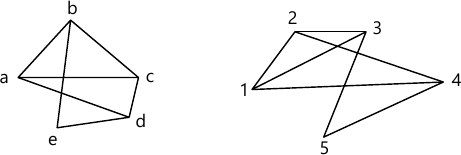MORE IN Discrete Structures
MU Computer Engineering (Semester 3)
Discrete Structures
December 2014
Total marks: --
Total time: --
INSTRUCTIONS
(1) Assume appropriate data and state your reasons
(2) Marks are given to the right of every question
(3) Draw neat diagrams wherever necessary

1 (a) Prove by mathematical induction xn-yn is divisible by x-y.
5 M
1 (b) How many vertices are necessary to construct a graph with exactly 6 edges in which each vertex is of degree 2.
5 M
1 (c) Show that a relation is reflexive and circular if and only if it is an equivalence relation.
5 M
1 (d) Prove that the set G={1,2,3,4,5,6} is an abelian group under multiplication modulo 7.
5 M

2 (a) Is it possible to draw a tree with five vertices having degrees 1,1,2,2,4?
4 M
2 (b) Find how many integers between 1 and 60 are
i) not divisible by 2 nor by 3 and nor by 5.
ii) Divisible by 2 but not by 3 and nor by 5.
8 M
2 (c) Solve the recurrence relation ar+2-ar+1-6a=4.
8 M

3 (a) Show that A?(B?C)=(A?B)?(A?C)
4 M
3 (b) State and explain Pigeonhole principle, extended Pigeonhole principle. How many numbers must be selected from the set {1,2,3,4,5,6} to guarantee that at least one pair of these numbers add up to 7?
8 M
3 (c) Let R be a relation on set A={1,2,3,4}, given as
R={(1,1), (1,4), (2,2), (2,3), (3,2), (3,3), (4,1), (4,4)}.
Find transitive closure using Warshall's Algorithm.
8 M

4 (a) Find the generating function for the following sequence
i) 1,2,3,4,5,6......
ii) 3,3,3,3,3......
4 M
4 (b) Show that the (2,5) encoding function e:B2?B5 defined by
e(00)=00000 e(01)=01110
e(10)=10101 e(11)=11011 is a group code.
How many errors will it detect and correct.
8 M
4 (c) Draw Hasse Diagram of D42. Find the complement of each element in D42.
8 M

5 (a) Define Distributive Lattice along with one appropriate example.
4 M
5 (b) Let the functions f,g and h defined as follows:
f:R→R, f(x)=2x+3
g:R→R, g(x)=3x+4
h:R→R, h(x)=4x
Find gof, fog, hof, gofoh
8 M
5 (c) $Let \ H= \begin{vmatrix} 1 &0 &0 \\0 &1 &1 \\1 &1 &1 \\1 &0 &0 \\0 &1 &0 \\0 &0 &1 \end{vmatrix}$ Be a parity check matrix. Determine the group code eH: B3 ? B6.
8 M

6 (a) Determine $[(p\Rightarrow q)\wedge q]\Rightarrow \neg p$ is a tautology.
4 M
6 (b) Define isomorphic graphs. Show that following graphs are isomorphic8 M
6 (c) R be a relation on set of integers Z defined by
R={(x,y)|x-y is divisible by 3}
Show that R is an equivalence relation and describe the equivalence classes.
8 M

More question papers from Discrete Structures